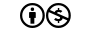# Python Quirks: Lambdas

The crippled nature of Python's lambdas are a big weakness of Python. They sit in a no-mans land of providing half a solution but being too crippled to actually to improve clarity / improve programming style.

Comments like this one about Python's lambdas are very, very, very common. Even Guido himself isn't particularly fond of lambdas, as demonstrated by his suggestion to remove them outright.

With all the negativity surrounding this admittedly underpowered language feature it is worth exploring why the capabilities of lambdas are so limited, and how to make use of them.

## History lesson

About 12 years ago, Python aquired acquired lambda, reduce(), filter() and map(), courtesy of (I believe) a Lisp hacker who missed them and submitted working patches.

Guido van Rossum (2005)

`lambda_input` was first introduced into the grammar on October 26th 1993. At this point `lambda` wasn't a keyword yet, but instead a function that would consume a `vararglist` (parameter list) and `testlist` (basically an expression) as a string.

```lambd​a('x: x + 1')
```

About one month later it finally became a keyword and has remained largely unchanged to this day.

## Pythonic?

For example, today someone claimed to have solved the problem of the multi-statement lambda.
But such solutions often lack "Pythonicity" [...]

Guido van Rossum (2006)

Technical feasibility is not really a concern when it comes to possible lambda improvements. The main issue is that Guido will not accept a solution that introduces indentation within expressions on the grounds of lacking "Pythonicity". This effectively rules out multi-statement lambdas.

```foo(
(
lambda a, b:
print(a)
print(b)
),
"bar",
)
```
```foo((
lambda a, b:
print(a)
print(b)
),
"bar",
)
```

If both variants were to be deemed valid, the concept of significant whitespace for indentation would be defeated, because the sparse syntax would require an optional extra level of indentation. If only one variant was to be allowed, there would be endless bike-shedding over which one should be chosen.

## Patterns

### Function binding

```from functools import partial

for fun in (partial(lambda number, exp: number ** exp, number) for number in range(RUNS)):
print(fun(2))
```

(Nested) lambdas can be used instead of `partial` for binding values to functions.

```for fun in ((lambda number: lambda exp: number ** exp)(number) for number in range(RUNS)):
print(fun(2))
```

Readability is questionable is both cases, but benchmarking (10^6*5 runs) reveals some noticeable speed improvements when using list comprehensions, which are eagerly evaluated. Generator expressions, which evaluate lazily, exhibit a smaller performance gain. The overhead of lambda binding is generally smaller, because a wrapper function is created instead of a `partial` object.

EagerLazy
PartialNestedImprovement
12.097278s11.102859s8,22%
12.075038s10.530266s12,80%
11.61805s10.08913s13,16%
PartialNestedImprovement
5.131468s4.854471s5,40%
4.223810s4.122884s2,39%
4.248887s4.158348s2,13%
Benchmark code
```from functools import partial
from time import time

ENTRIES = 5000000
RUNS = 2
FUNCTION_ARG = (2,)

def bench(generator_expression, expand):
start_bind = time()
callables_ = list(generator_expression) if expand else generator_expression
end_bind = time()

start_exec = time()

for fun in callables_:
fun(*FUNCTION_ARG)

end_exec = time()

return (end_bind - start_bind) if expand else None, (end_exec - start_exec)

def format_result(result, expand):
return "%sExecuting time: %fs\nOverall time: %fs" % (
"Binding time: %fs\n" % result if expand else "",
result,
(result + result) if expand else result,
)

for expand in (True, False):
print((" eager " if expand else " lazy ").center(20, "-"))
for _ in range(RUNS):
print(" partial ".center(20, "-"))
print(
format_result(
bench(
(
partial(lambda number, exp: number ** exp, number)
for number in range(ENTRIES)
),
expand,
),
expand,
)
)

print(" lambda ".center(20, "-"))
print(
format_result(
bench(
(
(lambda number: lambda exp: number ** exp)(number)
for number in range(ENTRIES)
),
expand,
),
expand,
)
)
```

### Computed sort key

```>>> from math import tan
>>> sorted(range(10, 20), key=lambda number: tan(number))
[11, 18, 15, 12, 19, 16, 13, 10, 17, 14]
```

## Conclusion

• List comprehensions are often faster and always more "Pythonic" than `map()`, `filter()`, and `reduce()`.
• Improving upon lambdas while preserving "Pythonicity" is hard.
• There are some legitimate use-cases for lambdas... just not that many.Attribution-NonCommercial 4.0 International (only applies to text, code license: MIT)